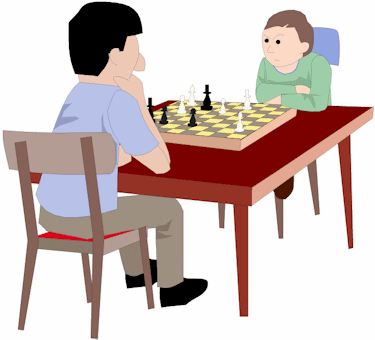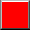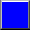This is a test to find out how much you know about THE VALUES OF THE PIECES. You have to get every question right to pass the test. So you need to be very careful and think very hard. Only your first answer counts. If you get a question wrong first time you can try again but you won't score a point.Q1. How many points is the PAWN worth?1 Point2 PointsQ2. How many points is the KNIGHT worth?2 Points3 PointsQ3. How many points is the BISHOP worth?3 Points5 PointsQ4. How many points is the ROOK worth?4 Points5 PointsQ5. How many points is the QUEEN worth?9 Points12 PointsQ6. We don't give the KING a value because it's worth the whole game.TrueFalseQ7. A BISHOP and PAWN together are worth more than a ROOK.TrueFalseQ8. A QUEEN and a PAWN are worth the same as TWO ROOKS.TrueFalseQ9. It's a fair exchange to swap a KNIGHT for a BISHOPTrueFalseQ10. A KNIGHT is worth more than TWO PAWNS.TrueFalseYou have now completed the VALUES OF PIECES quiz.What would you like to do next?Repeat the quizReturn to the menu